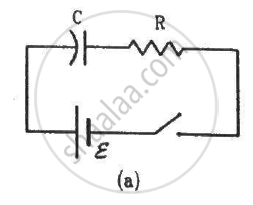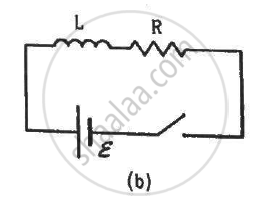Advertisement Remove all ads

# The Switches in Figure (A) and (B) Are Closed At T = 0 and Reopened After a Long Time At T = T0. - Physics

Sum

The switches in figure (a) and (b) are closed at t = 0 and reopened after a long time at t = t0.(a) The charge on C just after t = 0 is εC.
(b) The charge on C long after t = 0 is εC.
(c) The current in L just before t = t0 is ε/R.
(d) The current in L long after t = t0 is ε/R.

Advertisement Remove all ads

#### Solution

(b) The charge on C long after t = 0 is εC.
(d) The current in L long after t = t0 is ε/R.

The charge on the capacitor at time ''t'' after connecting it with a battery is given by,

Q=C epsilon[1-e^(-t"/RC")]

Just after t = 0, the charge on the capacitor will be

Q=C epsilon[1-e^0]=0

For a long after time, t → ∞

Thus, the charge on the capacitor will be

Q = Cepsilon[1-e^(-infty)]

rArr Q=Cepsilon[1-0]=Cepsilon

The current in the inductor at time ''t'' after closing the switch is given by

I=V_b/R(1-e^(-tR"/L"))

Just before the time t0, current through the inductor is given by

I=V_b/R(1-e^(-t_0R"/L"))

It is given that the time t0 is very long.

∴ t0 → ∞

I=epsilon/R(1-e^-infty)=epsilon/R

When the switch is opened, the current through the inductor after a long time will become zero.

Is there an error in this question or solution?
Advertisement Remove all ads

#### APPEARS IN

HC Verma Class 11, Class 12 Concepts of Physics Vol. 2
Chapter 16 Electromagnetic Induction
MCQ | Q 10 | Page 305
Advertisement Remove all ads

#### Video TutorialsVIEW ALL 

Advertisement Remove all ads
Share
Notifications

View all notifications

Forgot password?